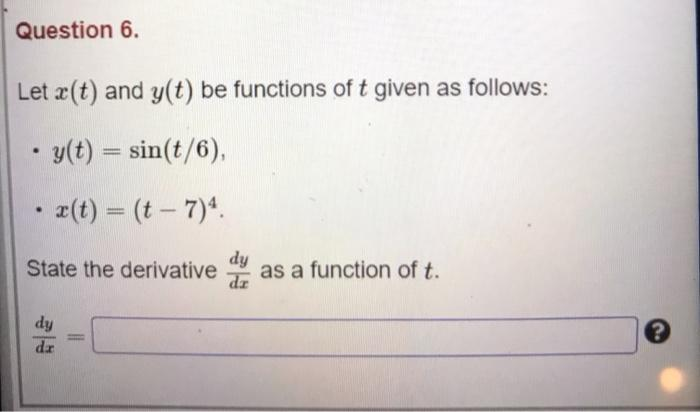### Create an Account

Already have account?

### Forgot Your Password ?

Home / Questions / Question 6. Let (t) and y(t) be functions of t given as follows: . y(t) = sin(t/6), • 2(t)...

# Question 6. Let (t) and y(t) be functions of t given as follows: . y(t) = sin(t/6), • 2(t) = (t – 7) State the derivative dy as a function of t. dy dir

Question 6. Let (t) and y(t) be functions of t given as follows: . y(t) = sin(t/6), • 2(t) = (t – 7) State the derivative dy as a function of t. dy dirApr 15 2021 View more View Less

#### Answer (Solved)Subscribe To Get Solution